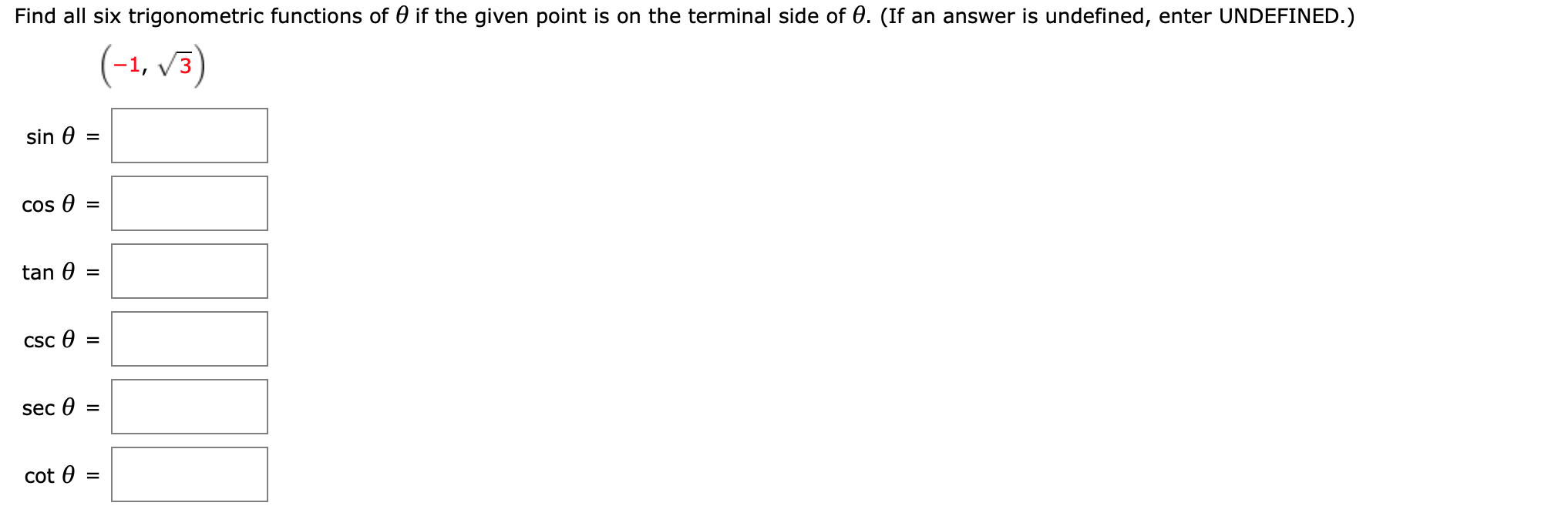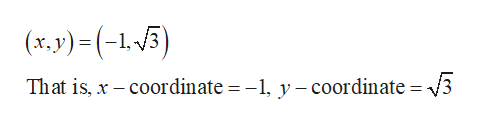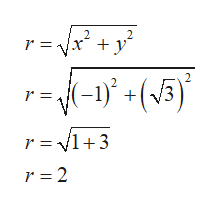Find all six trigonometric functions of 0 if the given point is on the terminal side of 0. (If an answer is undefined, enter UNDEFINED.)(-1, v3)sin ecos =tan 0CSCsec =cot 0

Question

find the 6 trig functionshelp_outlineImage TranscriptioncloseFind all six trigonometric functions of 0 if the given point is on the terminal side of 0. (If an answer is undefined, enter UNDEFINED.) (-1, v3) sin e cos = tan 0 CSC sec = cot 0 fullscreen
Step 1

From the  given point the value of the x-coordinate and y-coordinate can be obtained as shown :help_outlineImage Transcriptionclose(x,y)=(-1-5) That is, x coordinate = -1, y -coordinate = /3 fullscreen
Step 2

Obtain the value of r as shown below:help_outlineImage Transcriptionclose2 r = r r = v1+3 r 2 fullscreen
Step 3

Obtain the value of sine fun...

Want to see the full answer?

See Solution

Want to see this answer and more?

Our solutions are written by experts, many with advanced degrees, and available 24/7

See Solution
Tagged in

Trigonometric Ratios